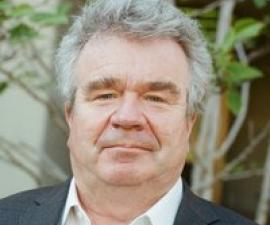### Research Expertise and Interest

mathematics, partial differential equations, mathematical physics, mathematical aspects of quantum mechanics, scattering theory, microlocal analysis